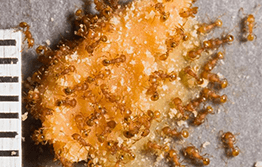```int(43)
bool(false)
int(0)
```
```int(1306)
bool(false)
int(43)
```
```int(2309)
bool(false)
int(43)
```
```int(55)
bool(false)
int(43)
```
```int(1307)
bool(false)
int(43)
```
```int(41)
bool(false)
int(0)
```
```int(60)
bool(false)
int(41)
```
```int(1311)
bool(false)
int(41)
```
```int(1371)
bool(false)
int(41)
```
```int(2576)
bool(false)
int(41)
```
```int(406)
bool(false)
int(41)
```
```int(42)
bool(false)
int(0)
```
```int(1304)
bool(false)
int(42)
```
```int(1303)
bool(false)
int(42)
```
```int(407)
bool(false)
int(42)
```
```int(62)
bool(false)
int(42)
```
```int(51)
bool(false)
int(42)
```
```int(2587)
bool(false)
int(42)
```
```int(3161)
bool(false)
int(42)
```
```int(3163)
bool(false)
int(42)
```
```int(3164)
bool(false)
int(42)
```
```int(3165)
bool(false)
int(42)
```
```int(3166)
bool(false)
int(42)
```
```int(3167)
bool(false)
int(42)
```
```int(3168)
bool(false)
int(42)
```
```int(3169)
bool(false)
int(42)
```
```int(3170)
bool(false)
int(42)
```
```int(3171)
bool(false)
int(42)
```
```int(3172)
bool(false)
int(42)
```
```int(3173)
bool(false)
int(42)
```
```int(3174)
bool(false)
int(42)
```
```int(2951)
bool(false)
int(0)
```
```int(2952)
bool(false)
int(2951)
```
```int(2953)
bool(false)
int(2951)
```
```int(2954)
bool(false)
int(2951)
```
```int(2955)
bool(false)
int(2951)
```Electric ants are regarded as one of the world’s 100 worst invasive species and are a serious environmental and urban pest. They can impact on the horticulture industry by painfully stinging employees and disrupt natural environments by damaging animals and plants. Domestic pets are at risk because the ants are attracted to pet food.

Electric ants are native to Central and South America. Adults are about 1.5mm long and ginger-coloured. They do not have a distinctive nest, but live under stones or wood, in dead trees and in houses, where they can infest furniture, beds and food.

It’s found in parts of Queensland. If you think you have seen electric ants, call 1800 084 881.Electric ants are spread by the movement of host materials such as timber, pot plants, rocks and tree limbs.